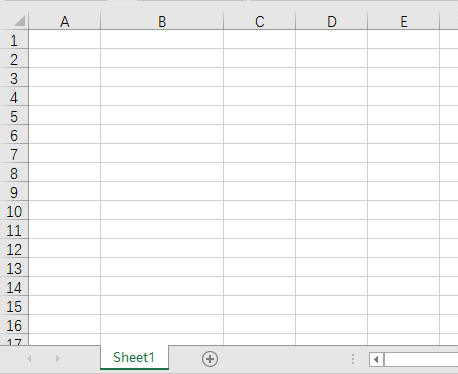### 小伙伴们好啊，今天老祝和大家一起分享一个记录数据录入时间的技巧。

Ctrl Shift ；（分号）

Ctrl ；（分号）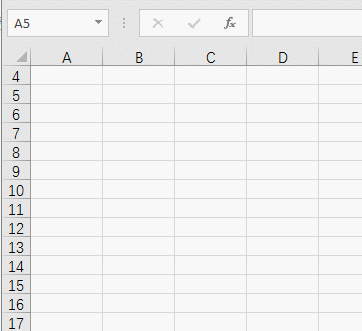=NOW()

=\$F\$1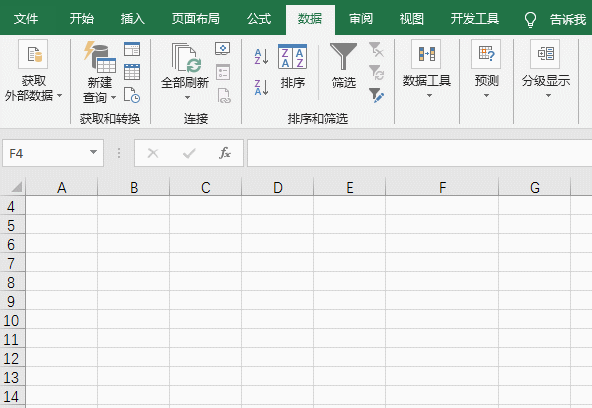e-mm-dd h:mm:ss

e-mm-dd h:mm:ss

=IF(C3="","",IF((C3=CELL("contents"))*(CELL("col")=3)*(CELL("row")=ROW()),NOW(),D3))

Cell函数用于返回活动单元格的信息，第一参数为"contents"时，返回活动单元格的内容。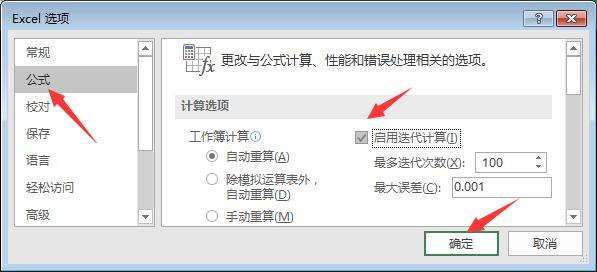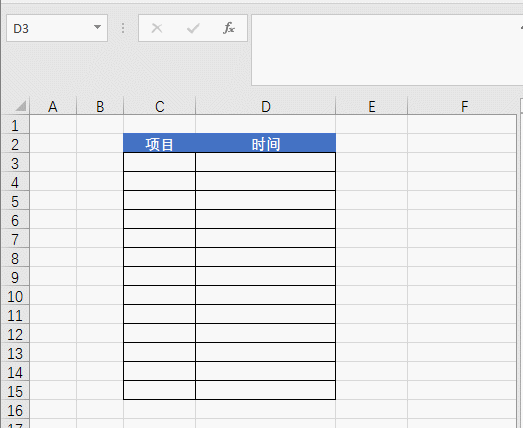`Private Sub Worksheet_Change(ByVal Target As Range)If Target.Column <> 1 Or Target.Row < 2 Then Exit SubTarget.Offset(0, 1).Value = NowEnd Sub`